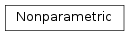# mvpa2.clfs.stats.Nonparametric¶class `mvpa2.clfs.stats.``Nonparametric`(dist_samples, correction='clip')

Non-parametric 1d distribution – derives cdf based on stored values.

Introduced to complement parametric distributions present in scipy.stats.

Methods

 `cdf`(x) Returns the cdf value at `x`. `fit`(dist_samples) `rcdf`(x) Returns cdf of reversed distribution (i.e.
Parameters: dist_samples : ndarray Samples to be used to assess the distribution. correction : {‘clip’} or None, optional Determines the behavior when .cdf is queried. If None – no correction is made. If ‘clip’ – values are clipped to lie in the range [1/(N+2), (N+1)/(N+2)] (simply because non-parametric assessment lacks the power to resolve with higher precision in the tails, so ‘imagery’ samples are placed in each of the two tails).

Methods

 `cdf`(x) Returns the cdf value at `x`. `fit`(dist_samples) `rcdf`(x) Returns cdf of reversed distribution (i.e.
`cdf`(x)

Returns the cdf value at `x`.

static `fit`(dist_samples)
`rcdf`(x)

Returns cdf of reversed distribution (i.e. if integrating from right tail)

Necessary for hypothesis testing in the right tail. It is really just a 1 - cdf(x) + pmf(x) == sf(x)+pmf(x) for a discrete distribution